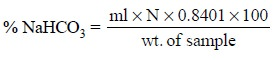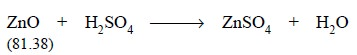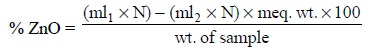Home | | Pharmaceutical Drug Analysis | Aqueous Titrations: Assay of Drugs

# Aqueous Titrations: Assay of Drugs

A few typical examples of acidimetric titrations, employing ‘direct titration method’ (DTM) and ‘residual titration method’ (RTM) from the ‘Pharmacopoeia of India’ are described here :

ASSAY OF DRUGS

A few typical examples of acidimetric titrations, employing ‘direct titration method’ (DTM) and ‘residual titration method’ (RTM) from the ‘Pharmacopoeia of India’ are described here :

## 1. DIRECT TITRATION METHOD

### 1.1. Sodium Carbonate (DTM)

Materials Required : 1 g of sodium carbonate ; 0.5 N sulphuric acid.

Procedure : Weigh accurately about 1 g, dissolve in 20 ml of water (DW) and titrate with 0.5 N sulphuric acid, using methyl orange solution as indicator. Each ml of 0.5 N sulphuric acid is equivalent to 0.42 g of NaHCO3.

Equation :

2NaHCO3 + H2SO4   → Na2SO4 + 2H2O + 2CO2

2(84.01)

It is evident from the above equation that 2 ml of NaHCO3 is equivalent to 1 ml of H2SO4. Hence, 1 ml of NaHCO3 is 1 equivalent and the equivalent weight is equal to the gram-molecular weight i.e., 84.01/ 2 = 42.0 g. One millilitre of 0.05 N sulphuric acid or 1 milliequivalent is equivalent to 42.0 mg or 1 meq of NaHCO3.

Thus, the purity of the sample assayed may be calculated as follows :### 1.2. Sodium Hydroxide (DTM)

Materials Required : 1.5 g of sodium hydroxide ; 1 N sulphuric acid.

Procedure : Weigh accurately about 1.5 g of sodium hydroxide and dissolve in about 40 ml of carbon-dioxide free distilled water (i.e., boiled and cooled DW). Cool and titrate with 1 N sulphuric acid using phenolphthalein solution as indicator. When the pink colour of the solution is discharged record the volume of acid solution required.

Equations : Thus, the end-point obtained with phenolphthalein as an indicator designates complete neutralization of all the NaOH as shown by the equation :........................(a)

However, in a cold solution, with phenolphthalein as an indicator, the end-point of titration of sodium carbonate with 1 N sulphuric acid is exhibited when the sodium carbonate is fully transformed into sodium carbonate, thus :...............................(b)

At this juncture, add methyl orange solution and proceed ahead with the titration until a persistant pink colour is produced. Each millilitre of 1 N sulphuric acid is equivalent to 0.040 g (or 40 mg) of total alkali, calculated as NaOH and each millilitre of acid consumed in the titration with methyl orange is equiva-lent to 0.106 g of Na2CO3.

Explanation

1. Titration to a phenolphthalein end-point serves two purposes :

(a) Neutralization of sodium hydroxide, and

(b) Conversion of Na2CO3 to NaHCO3.

2. When the Na2co3 is converted to NaHCO3 ( CO32-+H+ < == > HCO3- )the H+ remains low because the CO 2– is strongly basic, thereby the pH of the resultng mixture ranges between 8 to 9.8. This is when phenolphthalein changes colour till the conversion of Na2CO3 to NaHCO3 is complete.

3. The HCO –, is weakly basic in nature due to : HCO + H+  + < == >  H CO , and the NaHCO3 thus formed remains unneutralised even though H+ has been increased to the point where phenolphthalein affords a change in colour.

4. The neutralization of the generated NaHCO3 is complete only when H+ has been enhanced by further addition of acid, as observed by the change in colour of methyl orange at pH 3.2 to 4.4.

The reaction is represented by the equation :Calculations : The total volume of 1 N sulphuric acid consumed in the titration was required to neutralize NaOH and Na2CO3, thereby converting the latter first to NaHCO3 at the phenolphthalein end-point and then to H2CO3 at the methyl orange end-point.

From Eq. (a), it may be observed that the equivalent weight of NaOH is 40.00 g. Hence, each millili-tre of the total amount of 1 N sulphuric acid consumed is equivalent to 40.00 mg or 1 meq of NaOH. Thus, the total alkalinity calculated as NaOH is therefore :The volume of 1 N sulphuric acid i.e., the difference between the acid consumed to a methyl orange end-point and the acid consumed to a phenolphthalein end-point, required to neutralize the NaHCO3 as in Eq. (c) is equal to the volume needed to generate the NaHCO3 from Na2CO3 as in Eq. (b). Thus, from Eq. (b) it may be calculated that each millilitre of 1 N sulphuric acid is equivalent to 106.0 mg of Na2CO3. Hence, the quantity (%) of Na2CO3 present in the sample is given by :### 1.3. Cognate Assays

Sodium bicarbonate ; sodium salicylate tablets## 2. RESIDUAL TITRATION METHOD

### 2.1. Zinc Oxide (RTM)

Materials Required : 1.5 g of zinc oxide ; 1 N sulphuric acid ; 1 N sodium hydroxide ; 2.5 g ammo-nium chloride.

Procedure : 1.5 g of freshly ignited and cooled zinc oxide is accurately weighed and dissolved with 2.5 g of ammonium chloride in 50 ml of 1 N sulphuric acid with the help of gentle heating. After complete dissolution, add methyl orange and titrate the excess of sulphuric acid with 1 N sodium hydoxide. Each millilitre of 1 N sulphuric acid is equivalent to 40.6 mg of ZnO.

Equation :The requisite quantity of ZnO gets dissolved in the sulphuric acid thereby neutralizing an equivalent amount as shown by the above equation. Thus, the amount of sulphuric acid neutralized by the ZnO is estimated by subtracting, from the total amount of sulphuric acid utilized, the quantity neutralized by the standard NaOH in the back titration. The equivalent weight of ZnO, as shown in the above equation comes out to be 40.69 g (i.e., 81.38/2 = 40.69). Hence, each millilitre of 1 N sulphuric acid, 1 meq neautralized by the ZnO, is equivalent to 40.68 mg or 1 meq of ZnO.

Thus, the percentage of zinc oxide present in the sample may be calculated as follows :### 2.2. Cognate Assays

Calamine ; Ephedrine ; Lithium carbonate ; Milk of Magnesia ; Magnesium stearate ; Sodium lactate Injection.Study Material, Lecturing Notes, Assignment, Reference, Wiki description explanation, brief detail
Pharmaceutical Drug Analysis: Aqueous Titrations : Aqueous Titrations: Assay of Drugs |

Related Topics